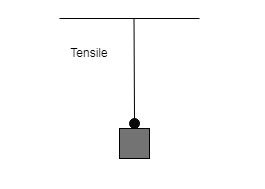# Tensile Stress

## Introduction

It is the quantity that is related to the stretching or in this case the tensile forces. This is responsible for the lengthening of a particular material along the course of the axis on which the load is applied. The tensile stress thus is associated with the magnitude of the force that is being applied upon a rod that has a certain extent of elasticity and the magnitude is divided by the cross-sectional area which is perpendicular to the force that is being applied onto the material. The tensile is a subdivision of normal stress that is a force that acts perpendicularly over a certain extent of the area of a given body and then the force is found to be spread across the unit area of that particular body.

## What is Tensile Stress?

Tensile stress is a subdivision of normal stress. This stress comes into existence when a force or load is applied that leans to stretch the material in the direction of the force.## What are the types of Stress?

Stress is defined as the reinstating force of the area per unit of the object. Stress is referred to as a scalar quantity which is denoted by $\mathrm{\sigma}$. In other words, stress is illustrated as the force quantity that is applied to material dissected by the area of crosssection of the material on which the force performs (Liu et al. 2020).

The reinstating force possesses a similar magnitude as the applied deforming force and may be in a contrasting direction. Stress can be expressed as $\mathrm{\sigma}$ = F / A, where F is force and A is the area of cross-section.

The stress is calculated in Pascal or N/m$\mathrm{^{2}}$. There are several types of stress in physics; however, normal stress, Tensile stress, shearing stress and longitudinal stress are the main classification of stress.

• Normal stress − The normal force is explained as the stress that increases when a force of the axis is applied to an element. The transmission of stress to the body is referred to as perpendicular (Wang et al. 2020). The length and volume of the object are changed and the stress level of the object returns to normal.

• Longitudinal stress − The changes in the body length because of normal stress are mentioned as longitudinal stress. This type of stress compresses or stretches the material throughout the complete length.

• Shearing stress − In this type of stress, the pressure is put tangentially across the surface area of the plane. The surface is termed to be tangent when the stress performing on the surface stretches a tangent and pressure operating on a surface is parallel.

• Tensile stress − Tensile stress is illustrated as the stress that happens when applied or disfiguring force causes a rise in the length of an object (Liao, Zhu, & Tang, 2019). For instance, opposite and equal forces are put at either side when a wire or rod is stretched.

## Difference between Tensile Stress and Compressive Stress

Tensile stress Compressive stress
It resulted in elongation of the object or particular happening. It is the consequence of the shortening of force applied.
It occurs by stretching forces. It occurs by Compressive forces.
Crane cable, ropes, nails and threads are the instances of tensile strength. Concrete pillars are the main instance of compressive stress.
Steel is the most powerful material under Tensile stress. Concrete and steel are the strongest under compressive stress.

## Application of Tensile Stress

Tensile stress is utilised by the movers and police to tow the vehicles and cars. Tensile stress works on Pulling of water where a pulley and rope create to bring up the liquid possible (Kim, Kim & Yoo, 2019).

It is seen when an individual sees the other pulling up the liquid from the well and taking it to the respective place.

Images Coming soon

Tensile stress works on a crane when it pulls up the materials to keep them somewhere else. Tug of war is the playing activity which used to work on the principle of Tensile stress (Wang et al. 2020). More applications of Tensile stress are playing of children, and Utilisation of weighing machines by vendors and shopkeepers to calculate the weight. The principle of Tensile stress works properly on anything where the force is applied in doing the work.

## Conclusion

The tensile stress is associated with the subdivision of the normal stress. The tensile stress is the relative force that is ting along the course of the axis of the given force that is accountable for the lengthening of the particular material. The magnitude of the applied force throughout the elastic rod is further sub-divided by the cross-sectional area of the rod. Furthermore, the magnitude of the force is perpendicular to the force that is benign applied.

## FAQs

Q.1. Which stresses can be calculated when a tensile force acts on a material?

Ans. Ultimate tensile stress is noted by the maximum stress that a material endures, modulus of resilience is the ratio between tensile stress and twice the young modulus of the material and facture stress, which is the maximum stress that the body can uphold in a crack point. All these aspects are calculated from the tensile force.

Q.2. Which substance has the highest tensile strength of all?

Ans. Tensile strength varies from one material to another. Multiwalled Carbon nanotubes has the highest tensile strength of all.

Q.3. What is the ultimate tensile stress (UTS)?

Ans. It is the maximum stress body can endure when force is applied. In case the force is applied beyond UTS, the material cracks.

Q.4. What is a major application of tensile stress?

Ans. Tensile stress is applied in various fields of work. It is used by police to tow cars and other vehicles.

Updated on: 09-Jan-2023

227 Views